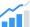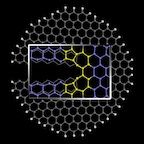# System Bits: Jan. 27

Analysis by MIT researchers shows how to get the best results when approximating solutions to complex engineering problems; Rice University researchers theorize that it should be possible to tune the properties of graphene.Optimizing algorithms
Optimization algorithms try to find the minimum values of mathematical functions, and are everywhere in engineering for such things as evaluating design tradeoffs, assessing control systems, finding patterns in data, among other things. A way to solve a difficult optimization problem is to first reduce it to a related but much simpler problem, then gradually add complexity back in, solving each new problem in turn and using its solution as a guide to solving the next one. This approach seems to work well in practice, but it’s never been characterized theoretically. Now, MIT researchers have described a way to generate a sequence of simplified functions that guarantees the best approximation that the method can offer.

To get a sense of how optimization works, suppose you’re a car designer trying to balance the costs of components made from different materials with the car’s weight and wind resistance, your function — known in optimization as a “cost function” — will be much more complex, but the principle is the same.

Machine-learning algorithms frequently attempt to identify features of data sets that are useful for classification tasks — say, visual features characteristic of cars. Finding the smallest such set of features with the greatest predictive value is also an optimization problem.

In essence, the new method begins by trying to find a convex approximation of an optimization problem, using a technique called Gaussian smoothing that converts the cost function into a related function that gives not the value that the cost function would, but a weighted average of all the surrounding values, which has the effect of smoothing out any abrupt dips or ascents in the cost function’s graph.

The weights assigned the surrounding values are determined by a Gaussian function, or normal distribution, while nearby values count more toward the average than distant values do.This sequence of graphs illustrates the application of the researchers’ technique to a real-world computer vision problem. The solution to each successive problem (red balls) is used to initialize (green arrows) the search for a solution to the next.
(Source: MIT)

The MIT researchers expect the practical utility of this to be something where there might be any number of different ways that you could go about doing smoothing or trying to do coarse-to-fine optimization; if one knows ahead of time that there’s a right one, then time is not wasted pursuing the wrong ones.

Tailoring graphene edges
Theoretical physicists at Rice University are studying the properties of graphene and figuring out how to fracture graphene nanoribbons to get the edges they need for applications.

They show it should be possible to control the edge properties of graphene nanoribbons by controlling the conditions under which the nanoribbons are pulled apart. The way atoms line up along the edge of a ribbon of graphene — the atom-thick form of carbon — controls whether it’s metallic or semiconducting. Current passes through metallic graphene unhindered, but semiconductors allow a measure of control over those electrons.

And since modern electronics are all about control, semiconducting graphene (and semiconducting 2D materials in general) are of great interest to scientists and industry working to shrink electronics for applications.Graphene nanoribbons can be enticed to form favorable “reconstructed” edges by pulling them apart with the right force and at the right temperature, according to researchers at Rice University. The illustration shows the crack at the edge that begins the formation of five- and seven-atom pair under the right conditions. (Source: Rice University)